International
Tables for
Crystallography
Volume B
Reciprocal space
Edited by U. Shmueli

International Tables for Crystallography (2006). Vol. B. ch. 2.1, pp. 199-200   | 1 | 2 |

## Section 2.1.7.2. Mathematical background

U. Shmuelia* and A. J. C. Wilsonb

aSchool of Chemistry, Tel Aviv University, Tel Aviv 69 978, Israel, and bSt John's College, Cambridge, England
Correspondence e-mail:  ushmueli@post.tau.ac.il

#### 2.1.7.2. Mathematical background

| top | pdf |

Suppose that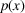is a p.d.f. which accurately describes the experimental distribution of the random variable x, where x is related to a sum of random variables and can be assumed to obey (to some approximation) an ideal p.d.f., say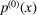, based on the central-limit theorem. In the correction-factor approach we seek to representas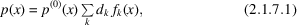where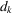are coefficients which depend on the cause of the deviation offrom the central-limit theorem approximation and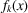are suitably chosen functions of x. A choice of the set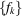is deemed suitable, if only from a practical point of view, if it allows the convenient introduction of the cause of the above deviation ofinto the expansion coefficients. This requirement is satisfied – also from a theoretical point of view – by takingas a set of polynomials which are orthogonal with respect to the ideal p.d.f., taken as their weight function (e.g. Cramér, 1951). That is, the functionsso chosen have to obey the relationship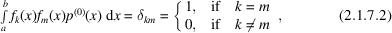where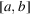is the range of existence of all the functions involved. It can be readily shown that the coefficientsare given by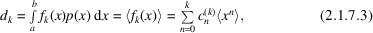where the brackets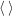in equation (2.1.7.3)denote averaging with respect to the unknown p.d.f.and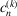is the coefficient of the nth power of x in the polynomial. The coefficientsare thus directly related to the moments of the non-ideal distribution and the coefficients of the powers of x in the orthogonal polynomials. The latter coefficients can be obtained by the Gram–Schmidt procedure (e.g. Spiegel, 1974), or by direct use of the Szegö determinants (e.g. Cramér, 1951), for any weight function that has finite moments. However, the feasibility of the present approach depends on our ability to obtain the moments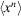without the knowledge of the non-ideal p.d.f.,.

### ReferencesCramér, H. (1951). Mathematical methods of statistics. Princeton University Press.Google ScholarSpiegel, M. R. (1974). Theory and problems of Fourier analysis. Schaum's Outline Series. New York: McGraw-Hill.Google Scholar